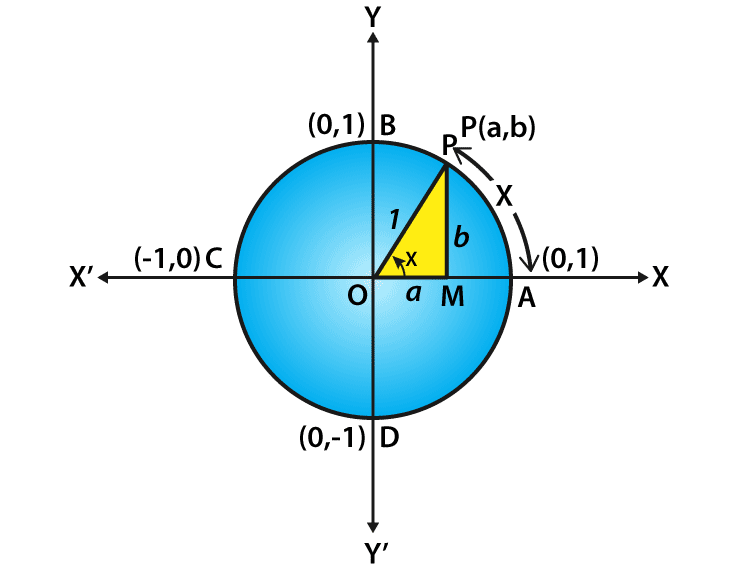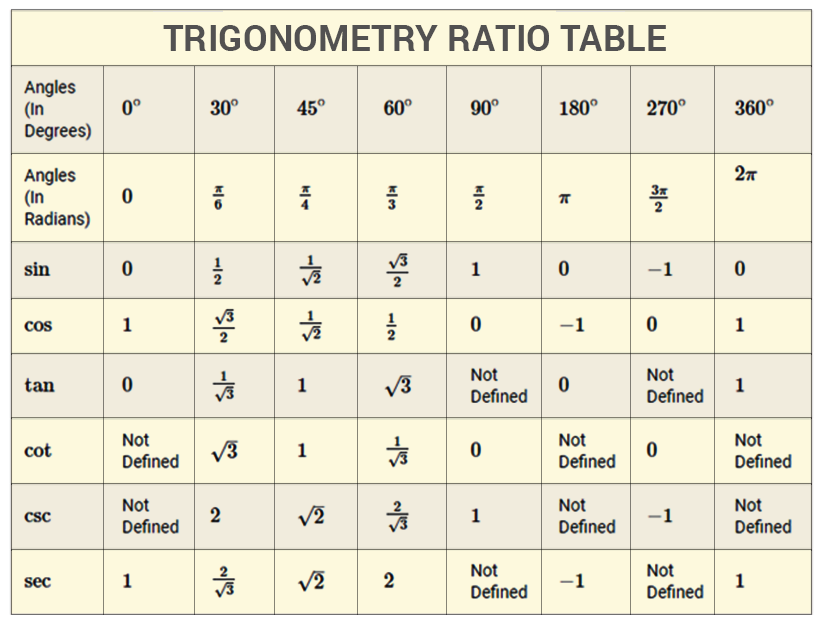# Cos 0

Here, we will discuss the value for cos 0 degrees and how the values are derived using the quadrants of a unit circle. The trigonometric functions are also known as an angle function that relates the angles of a triangle to the length of the triangle sides. Trigonometric functions are one of the most important topics which are used in the study of periodic phenomena like sound and light waves, the study of harmonic oscillators and finding the average temperature variations. The three familiar trigonometric ratios are sine function, cosine function, and tangent function. It is commonly defined for the angles less than a right angle, trigonometric functions are stated as the ratio of two sides of a right triangle containing the angle in which the values can be found in the length of various line segments around a unit circle. The angles of a triangle are calculated with respect to sin, cos and tan functions. Usually, the degrees are represented as 0°, 30°, 45°, 60°, 90°, 180°, 270° and 360°.

## Cos 0 Degree Value

To define the cosine function of an acute angle, start with the right-angled triangle ABC with the angle of interest and the sides of a triangle. The three sides of the triangle are defined as follows:

• The opposite side is defined as the side opposite to the angle of interest.
• The hypotenuse side is the side opposite the right angle and it should be the longest side of a right triangle
• The adjacent side is the remaining side where it forms a side of both the angle of interest and the right angle

The cosine function of an angle is equal to the length of the adjacent side divided by the length of the hypotenuse side and the formula is given by

Cos θ = Adjacent Side / Hypotenuse Side

## Value of Cos 0 Using Unit Circle

Assume a unit circle with the center at the origin of the coordinate axes. Consider that P (a, b) be any point on the circle which forms an angle AOP = x radian. This means that the length of the arc AP is equal to x. So we define that cos x = a and sin x = bNow consider a triangle OMP is a right triangle,

By using the Pythagorean theorem, we get

OM2+ MP2= OP2 (or) a2+ b2= 1

So for every point on the unit circle, we define it as

a2+ b2 = 1 (or) cos2 x + sin2 x = 1

It is noted that the one complete revolution subtends an angle of 2π radian at the centre of the circle,

∠AOB=π/2,

∠AOC = π and

∠AOD =3π/2.

Since all angles of a triangle are the integral multiples of π/2 and it is commonly called as quadrantal angles. Therefore, the coordinates of the points A, B, C and D are (1, 0), (0, 1), (–1, 0) and (0, –1) respectively. Therefore, from the quadrantal angles, we can get the cos 0 value

Cos 0° = 1

Now, when we take one complete revolution from the point P, again it comes back to the same point P. So, we also observe that the values of sine and cosine functions do not change, if x increases or decreases by an integral multiple of 2π,

cos (2nπ + x) = cos x, where n ∈ Z

Further, it is observed that

cos x = 0, when x = ±π/2, ±3π/2, ±5π/2, … It means that cos x vanishes when x is an odd multiple of π/2.

So, cos x = 0 implies x = (2n + 1)π/2 , where n takes the value of any integer.

For a triangle, ABC having the sides a, b, and c opposite the angles A, B, and C, the cosine law is defined.

Consider for an angle C, the law of cosines is stated as

c2 = a2 + b2– 2ab cos(C)

In the same way, we can derive other values of cos degrees like 30°, 45°, 60°, 90°, 180°, 270°and 360°. Also, it is easy to remember the special values like 0°, 30°, 45°, 60°, and 90° since all the values are present in the first quadrant. All the sine and cosine functions in the first quadrant take the form $\frac{\sqrt{n}}{2}$ or  $\sqrt{\frac{n}{4}}$. Once we find the values of sine functions it is easy to find the cosine functions.

Sin 0° = $\sqrt{\frac{0}{4}}$

Sin 30° = $\sqrt{\frac{1}{4}}$

Sin 45° = $\sqrt{\frac{2}{4}}$

Sin 60° = $\sqrt{\frac{3}{4}}$

Sin 90° = $\sqrt{\frac{4}{4}}$

Now Simplify all the sine values obtained and put in the tabular form:

 0° 30° 45° 60° 90° Sin 0 1/2 $\frac{1}{\sqrt{2}}$ $\frac{\sqrt{3}}{2}$ 1

From the values of sine, we can easily find the cosine function values. Now, to find the cos values, fill the opposite order the sine function values. It means that

Cos 0° = Sin 90°

Cos 30° = Sin 60°

Cos 45° = sin 45°

Cos 60° = sin 30°

Cos 90° = sin 0°

So the value of cos 0 degrees is equal to 1 since cos 0° = sin 90°

 0° 30° 45° 60° 90° Sin 0 1/2 $\frac{1}{\sqrt{2}}$ $\frac{\sqrt{3}}{2}$ 1 Cos 1 $\frac{\sqrt{3}}{2}$ $\frac{1}{\sqrt{2}}$ 1/2 0

In a similar way, we can find the values of other degrees of trigonometric functions depends on the quadrant value. Below is the trigonometry table, which defines all the values of cosine function along with other trigonometric ratios.### Example

Question:

Find the value of cos 15°

Solution:

Cos 15°= cos(45°-30°)

Now, take the values a = 45° and b = 30°

By using the formula, Cos (a-b) = cos a cos b + sin a sin b

So, it becomes Cos 15° = cos 45° cos 30° +sin 45° sin 30°

$\cos 15^{\circ}= \frac{1}{\sqrt{2}}\frac{\sqrt{3}}{2}+\frac{1}{\sqrt{2}}\frac{1}{2}$ $\cos 15^{\circ}= \frac{\sqrt{3}}{2\sqrt{2}}+\frac{1}{2\sqrt{2}}$ $\cos 15^{\circ}= \frac{\sqrt{3}+1}{2\sqrt{2}}$

For more information on cos 0 and other trigonometric functions, visit BYJU’S and also watch the interactive videos to clarify the doubts.

 Related Links Sin 0 Degree Trigonometry Formulas Inverse Cosine Cosine Rule